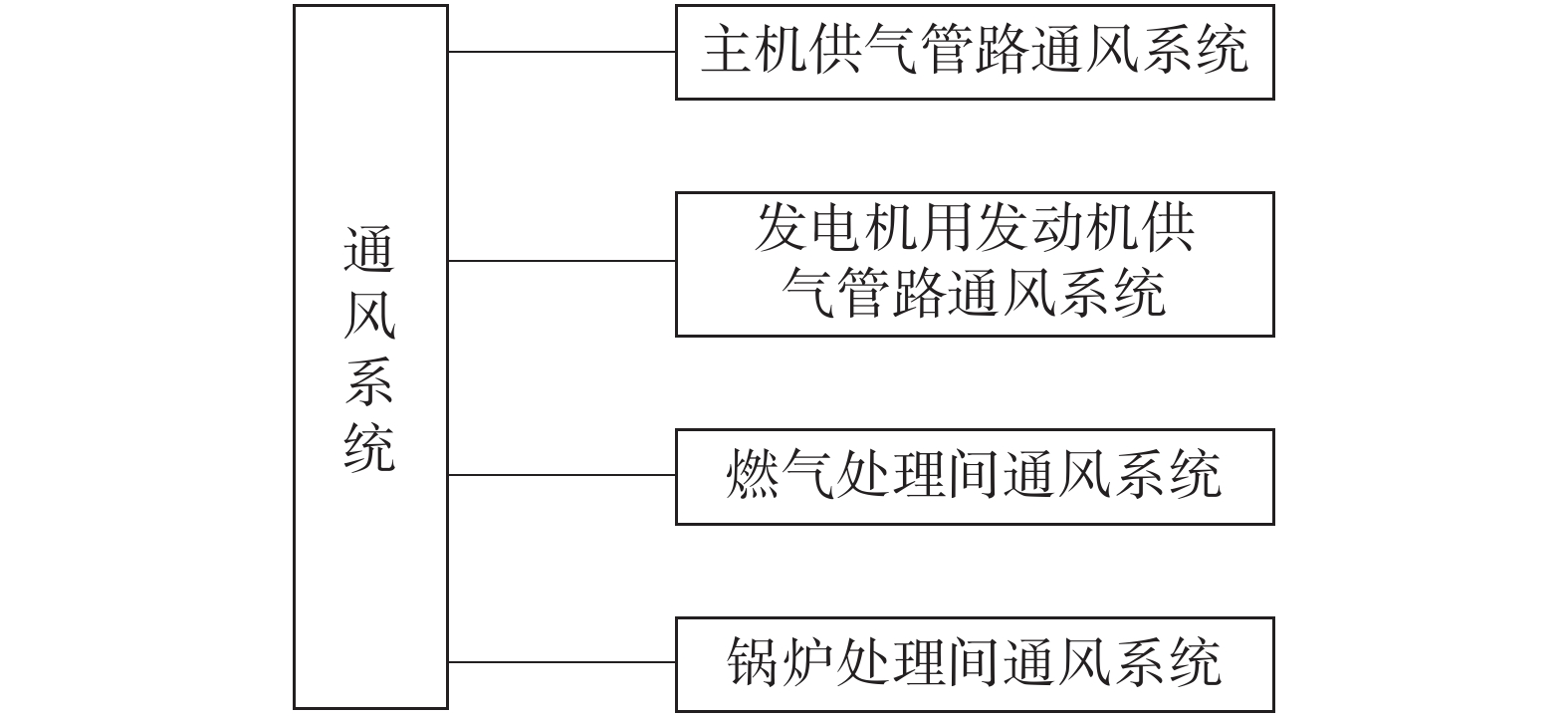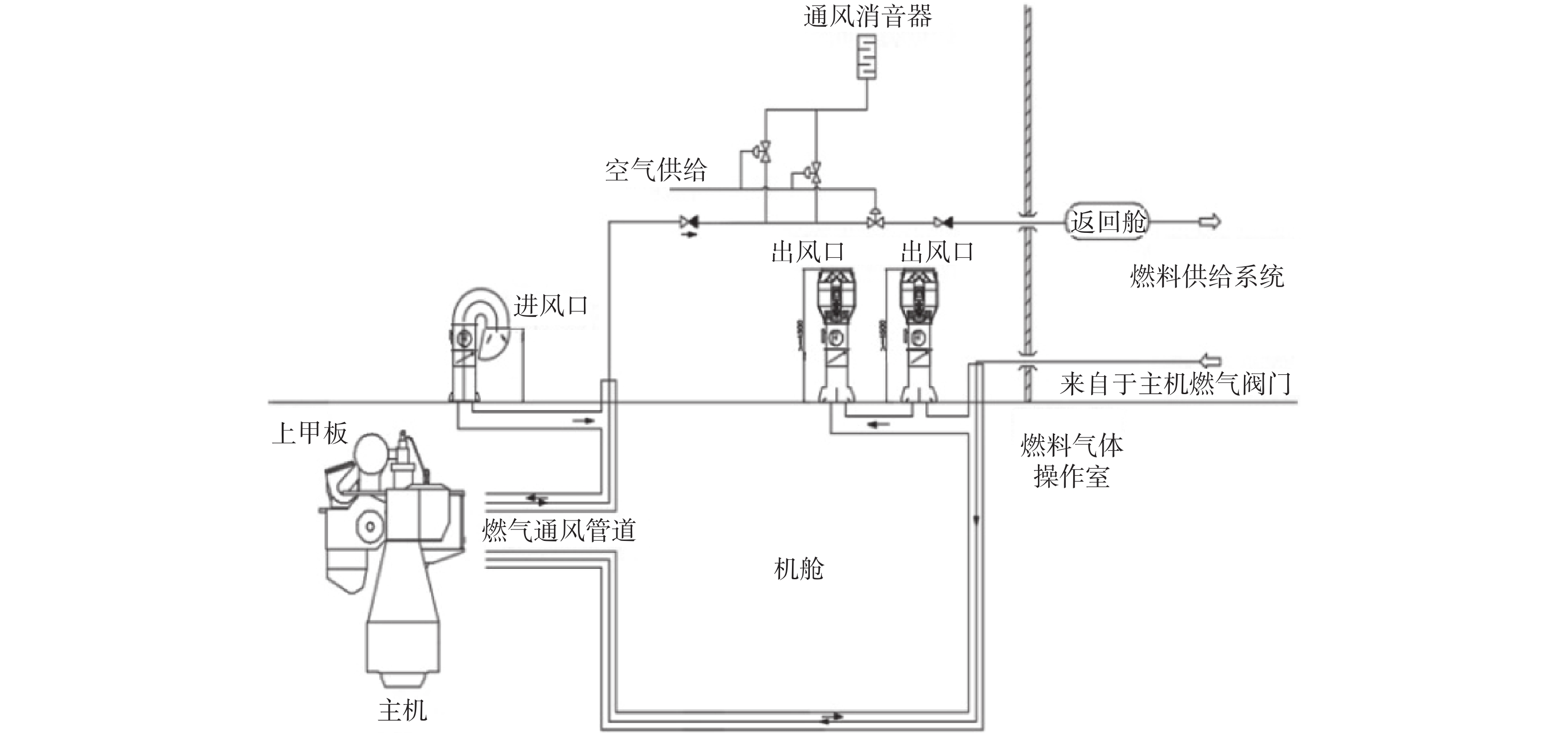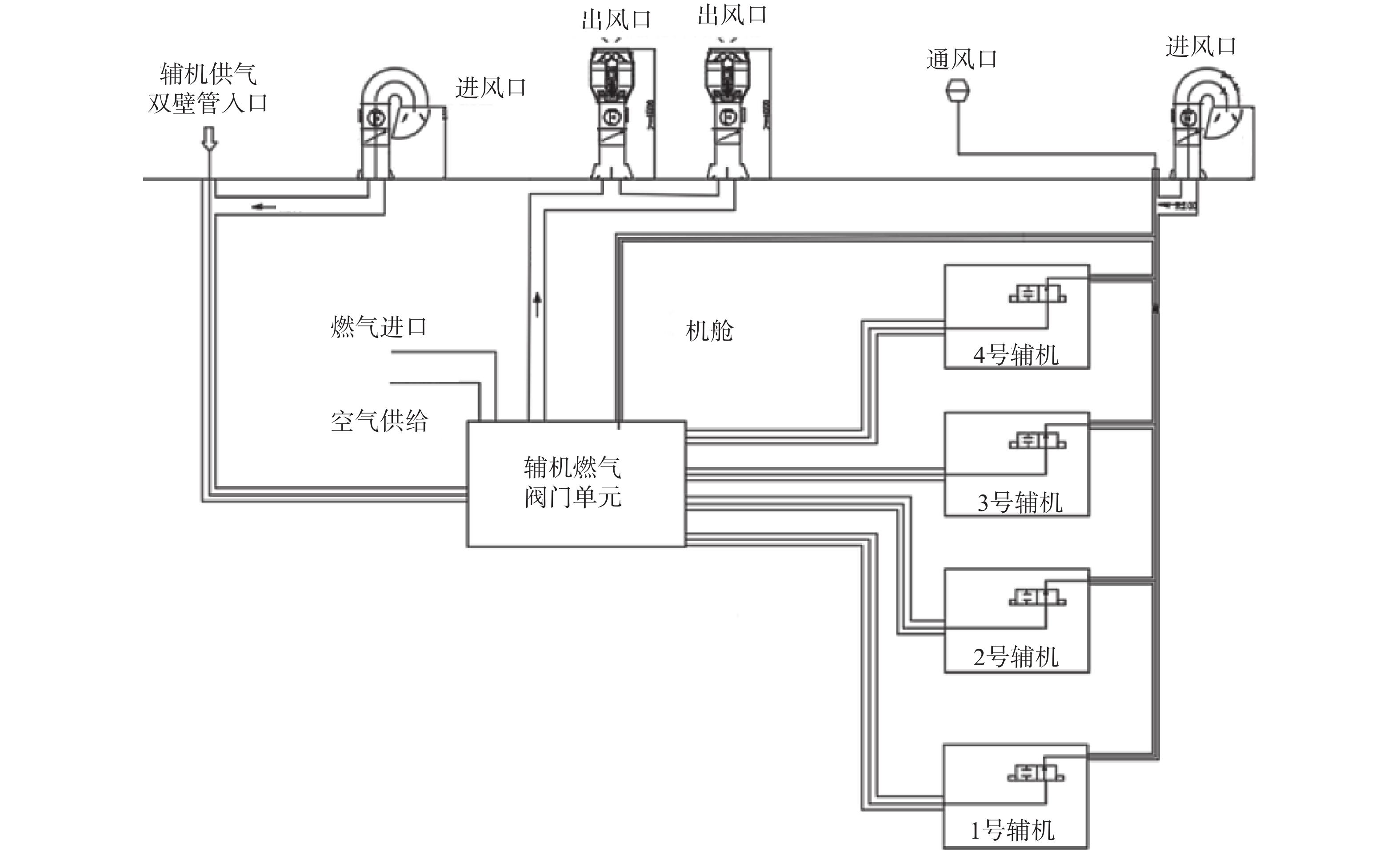﻿ 双燃料集装箱船机舱通风系统设计与配置
«上一篇文章快速检索 高级检索

 应用科技2019, Vol. 46Issue (3): 1-6  DOI: 10.11991/yykj.2018090090

### 引用本文GAO Aihua, MA Zhongli, LOU Chunjing, et al. Design of the ventilation system in the cabin of dual fuel large container ships[J]. Applied Science and Technology, 2019, 46(3), 1-6. DOI: 10.11991/yykj.201809009.### 文章历史

1. 上海外高桥造船有限公司，上海 200137;
2. 成都信息工程大学 控制工程学院，四川 成都 610225

Design of the ventilation system in the cabin of dual fuel large container ships
GAO Aihua 1 , MA Zhongli 2, LOU Chunjing 1 , KUAI Jingjing 1
1. Shanghai Waigaoqiao Shipbuilding Co. Ltd., Shanghai 200137, China;
2. School of Control Engineering, Chengdu University of Information Technology, Chengdu 610225, China
Abstract: For liquefied natural gas (LNG)/diesel dual fuel ships, accidental leakage and accumulation of explosive, flammable or toxic gases poses a significant risk to the safety of ships, personnel and equipment. In order to ensure that 20 000 tanks of LNG/diesel dual fuel ocean-going container ships can effectively discharge dangerous gases in relatively narrow cabin space, the cabin ventilation system of this type of ship is optimized and configured. Firstly, according to the requirements of the intrinsically safe cabin, the overall design scheme of the quality and intrinsically safety cabin ventilation system is given. Then, through the analysis and calculation of air consumption and ventilation, the calculation and fan selection of ventilation capacity are finished for the cabin, the main engine supply pipeline and its Gas Valve Unit, the generator pipeline and GVU, boiler piping and GVU, and gas processing room; finally, the configuration scheme of the main engine supply line, the generator supply line, and the corresponding GVU ventilation of the LNG fuel supply system are discussed. The analysis and calculation show that the designed and deployed ventilation system can not only provide enough air for the main equipments, but also control the amount of dangerous gas in a safe range to ensure the normal and safe operation of the ship.
Keywords: dual fuel    container ship    liquefied natural gas    engine room    intrinsically safty    ventilation system    fan    gas supply pipeline

1 LNG燃料供给系统通风设计和配置 1.1 本质安全型机舱

1.2 本质安全型机舱的通风设计 1.2.1 通风系统总体设计

1)危险场所的通风管道应与非危险场所的通风管道分开，且能在所有温度和环境条件下正常工作。

2)应能避免任何气体积聚，系统由独立风扇组成，要保证容量足够。

3)非危险空间的空气出口设在危险区域之外。

4)危险封闭空间的空气出口设在与通风空间相同或较小的危险程度区域内。

5)通风设备所需的容量要根据房间的总容积计算。对于复杂形式的房间，需要增加通风能力。

6)可通过入口由非危险区域进入危险区域的处所，应设置空气锁，并保持在相对于外部危险区域的超压状态。Download: 图 1 通风系统整体构成
1.2.2 主机供气管路通风系统设计

1.2.3 辅机供气管路通风系统设计

1.2.4 燃气处理间通风系统设计

1.2.5 锅炉管路通风系统设计

2 LNG燃料供给系统风机容量计算选型

2.1 机舱通风容量计算及风机选型表 1 通风系统计算参数依据

2.1.1 机舱燃烧耗气量计算

1)推进柴油机燃烧空气量qdp计算

 ${q_{{\rm{dp}}}} = \frac{{{P_{{\rm{dp}}}}{m_{{\rm{ad}}}}}}{\rho } = \frac{{55 \; 000 \times {\rm{0}}.{\rm{002}} \; {\rm{3}}}}{{{\rm{1}}.{\rm{13}}}} = 111.94 \; {{\rm{m}}^{\rm{3}}}/{\rm{s}}$

2)发电用柴油机燃烧空气量qdg计算

 ${q_{{\text{dg}}}} = \frac{{{P_{{\text{dg}}}}K{m_{{\text{ad}}}}}}{\rho }n = \frac{{4 \; 500 \times {\text{80}}\% \times {\text{0}}.{\text{002}}}}{{{\text{1}}.{\text{13}}}} \times {\text{3}} = 19.11 \; {{\text{m}}^{\text{3}}}/{\text{s}}$

3)锅炉燃烧空气量计算

 ${q_{{b}}} = \frac{{{G_{{b}}}{m_{{\rm{fs}}}}{m_{{\rm{af}}}}}}{\rho } = \frac{{\displaystyle\frac{{6 \times {\rm{1}} \; {\rm{000}}}}{{{\rm{3}} \; {\rm{600}}}} \times {\rm{0}}.{\rm{075}} \times {\rm{15}}.{\rm{7}}}}{{{\rm{1}}.{\rm{13}}}} = {\rm{1}}.73 \; {{\rm{m}}^{\rm{3}}}/{\rm{s}}$

4)燃烧所需总空气量计算

 ${q_c} = {q_{{\rm{dp}}}} + {q_{{\rm{dg}}}} + {q_{{b}}} + {q_{{a}}} = 132.85 \; {{\rm{m}}^{\rm{3}}}{\rm{/s}}$

2.1.2 机舱散热耗气量计算

1)推进柴油机散热量计算

 ${\mathit{\Phi}_{{\rm{dp}}}} = {\rm{0}}.{\rm{141}}{{\ P}_{{\rm{dp}}}}^{{\rm{0}}.{\rm{26}}} = {\rm{0}}.{\rm{141}} \times 55 \; {000^{{\rm{0}}.{\rm{76}}}} = 564.8 \; {\rm{kW}}$

2)发电用柴油机散热量计算

 \begin{aligned} {\phi _{{\text{dg}}}} = & 0.396{({P_{{\text{dg}}}}K)^{0.7}}n = 0.396 \times \\ & {\left( {4\;500 \times 80\% } \right)^{0.7}} \times 3 = 366.6 \; {\text{kW}} \\ \end{aligned}

3)蒸汽锅炉散热量计算

 \begin{aligned} {\mathit{\Phi}_{{b}}} = & {m_{\text{S}}}{m_{{\text{fs}}}}h\frac{{\Delta {h_{{b}}}}}{{{\text{100}}}}{B_{\text{1}}} = \frac{{6 \times 1 \; 000}}{{3 \; 600}} \times 0.077 \times \\ & 40 \; 200 \times \frac{{80\% }}{{100}} \times 1 = 41.2 \; {\text{kW}} \\ \end{aligned}

4)蒸汽管和凝水管散热量计算

 ${\varPhi _{{\rm{ps}}}} = {m_{{\rm{sc}}}}\frac{{\Delta {h_p}}}{{{\rm{100}}}} = \frac{{1 \;200}}{{1.6}} \times \frac{{20\% }}{{100}} = 1.5 \; {\rm{kW}}$

5)发电机散热量计算

 \begin{aligned} {\varPhi _{{g}}} = & {P_{{g}}}K({\text{1}} - \eta )n = 4\;150 \times {\text{0}}.{\text{80}} \times \hfill \\ & ({\text{1}} - {\text{94}}\% ) \times {\text{3}} = 597 \; {\text{kW}} \hfill \\ \end{aligned}

6)电气设备的散热量计算

 ${\varPhi _{{\rm{el}}}} = {\rm{100}} \; {\rm{kW}}$

7)排气管和废气锅炉散热量计算

 ${\varPhi _{{\rm{pg}}}} = 10 \; {\rm{kW}}$

8)加热箱柜散热量计算

 ${\varPhi _{{t}}} = 2 \; {\rm{kW}}$

9)机舱中其他设备发热量估算值计算

 ${\varPhi_{\rm{0}}} = {\rm{4}}0 \; {\rm{kW}}$

10)所有设备散热所需通风量qh计算

 ${q_{{h}}} = \frac{{\Sigma \varPhi }}{{\rho {{c}}\Delta T}} - {\rm{0}}.{\rm{4}}({q_{{\rm{dp}}}} + {q_{{\rm{dg}}}}) - {q_{{b}}} = 66.63 \; {{\rm{m}}^{\rm{3}}}{\rm{/s}}$

2.1.3 机舱通风总量及风机选型

1)机舱通风总量计算

 \begin{aligned} Q = & {q_c} + {q_h} = 132.85 + 66.63 = \\ & 199.48 \; {{\text{m}}^{\text{3}}}{\text{/s}} = 718 \; 128 \; {{\text{m}}^{\text{3}}}{\text{/h}} \end{aligned}

 ${Q_{{\rm{each}}}} = 718 \; 128/6 = 119 \; 688 \; {{\rm{m}}^{\rm{3}}}{\rm{/h}}$

2)机舱送风机选型表 2 机舱送风机选型参数
2.2 主机供气管路及GVU通风容量计算及风机选型

1)主机供气管路及GVU通风总量计算

 ${Q_{{\rm{pr}}}} = {T_{{\rm{pr}}}}{V_{{\rm{pr}}}} = 12.8 \times 30 = 384 \; {{\rm{m}}^{\rm{3}}}{\rm{/h}}$

2)主机供气管路抽风机选型表 3 主机供气管路抽风机选型参数
2.3 发电机管路及GVU通风容量计算及风机选型

 ${Q_{{\rm{pr}}}} = {T_{{\rm{pr}}}}{V_{{\rm{pr}}}} = 2.7 \times 30 = 81 \; {{\rm{m}}^{\rm{3}}}{\rm{/h}}$

 ${Q_{{\rm{pr}}}} = 81 \times {\rm{2 = 162}} \;{{\rm{m}}^{\rm{3}}} {\rm{/h}}$表 4 发电机管路抽风机选型参数
2.4 锅炉管路及GVU通风容量计算及风机选型

 ${Q_{{\rm{pr}}}} = {T_{{\rm{pr}}}}{V_{{\rm{pr}}}} = 0.6 \times 30 = 18 \; {{\rm{m}}^{\rm{3}}}{\rm{/h}}$表 5 锅炉抽风机选型参数
2.5 燃气处理间通风容量计算及风机选型

 ${Q_{{\rm{pr}}}} = {T_{{\rm{pr}}}}{V_{{\rm{pr}}}} = 1 \; 933 \times 30 = 57 \; 984 \; {{\rm{m}}^{\rm{3}}}{\rm{/h}}$表 6 燃气处理间抽风机选型参数
3 LNG燃料供给系统通风布置

3.1 主机供气管路及GVU通风布置Download: 图 2 主机供气管路通风管系走向图

3.2 发电机供气管路及GVU通风布置Download: 图 3 辅机供气管路及GVU通风管系走向

4 结论

1)设计的双燃料船本质安全型机舱通风系统符合相关规范要求，能够保证危险气体的有效可靠排出，保障船舶双燃料的可靠安全使用；

2)本文设计以工程可实现性为出发点，设计的通风系统较易工程实现；

3)本文设计的针对双燃料集装箱船机舱的通风系统为其他LNG燃料系统的通风系统的设计提供了设计思路和依据。

  徐博. 世界LNG发展现状与趋势[J]. 北京石油管理干部学院学报, 2004, 11(2): 9-13. (0)  GUAN Yifeng, ZHAO Jie, SHI Tengfei, et al. Fault tree analysis of fire and explosion accidents for dual fuel (diesel/natural gas) ship engine rooms[J]. Journal of marine science and application, 2016, 15(3): 331-335. DOI:10.1007/s11804-016-1366-6 (0)  宁宝东. 船舶机舱通风和风机的选型[J]. 船舶, 2001(5): 62-63. DOI:10.3969/j.issn.1001-9855.2001.05.015 (0)  裘继承. 拖网渔船LNG单燃料动力系统的设计[J]. 广东造船, 2014(4): 75-77. DOI:10.3969/j.issn.2095-6622.2014.04.025 (0)  何晓聪, 周瑞平. LNG燃料动力船机舱设计研究[J]. 造船技术, 2013(6): 9-13. DOI:10.3969/j.issn.1000-3878.2013.06.003 (0)  中国船级社武汉规范研究所. 天然气燃料动力船舶规范[J]. 船舶标准化工程师, 2014, 47(1): 87-93. (0)  许超逸. 船舶机舱火灾特性的数值模拟研究[D]. 哈尔滨: 哈尔滨工业大学, 2016. (0)  刘秀丽, 孙琦, 柳国胜, 等. LNG燃料推进散货船的开发技术[J]. 船舶标准化工程师, 2014, 47(1): 9-13,44. DOI:10.3969/j.issn.1005-7560.2014.01.003 (0)  吴吓华. 探讨火电厂中锅炉运行优化设计[J]. 中国新技术新产品, 2014(22): 70. DOI:10.3969/j.issn.1673-9957.2014.22.056 (0)  海安会MSC.370(93)决议. 《国际散装运输液化气体船舶构造和设备规则》IGC中文版[EB/OL]. [2018−08−20]. (2014−05−22). https://wenku.baidu.com/view/12bfb9ebdbef5ef7ba0d4a7302768e9951e76e94.html. (0)  孙成琪, 安连彤, 林安平. 3600 PSASD拖轮通风装置选型设计及CO2流量计算 [J]. 造船技术, 2013(3): 15-18. DOI:10.3969/j.issn.1000-3878.2013.03.004 (0)  中国船级社. 双燃料发动机系统设计与安装指南[EB/OL]. [2018−06−26]. (2016−01−18). https://max.book118.com/html/2016/0118/33503640.shtm (0)